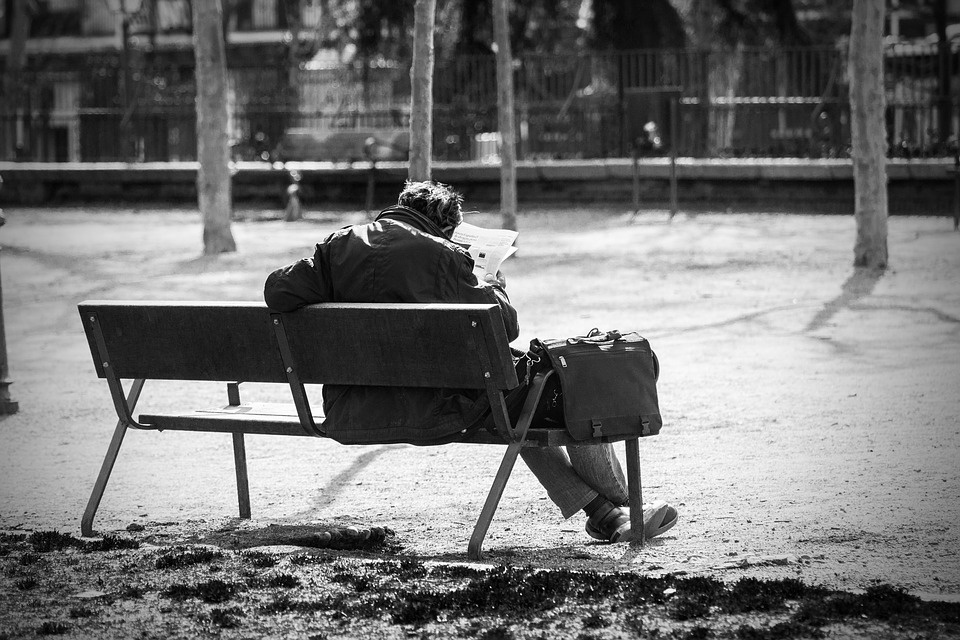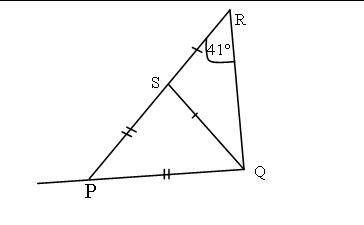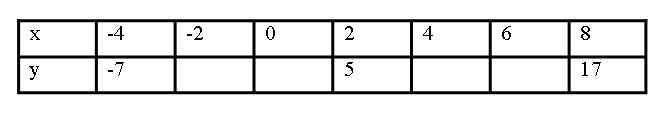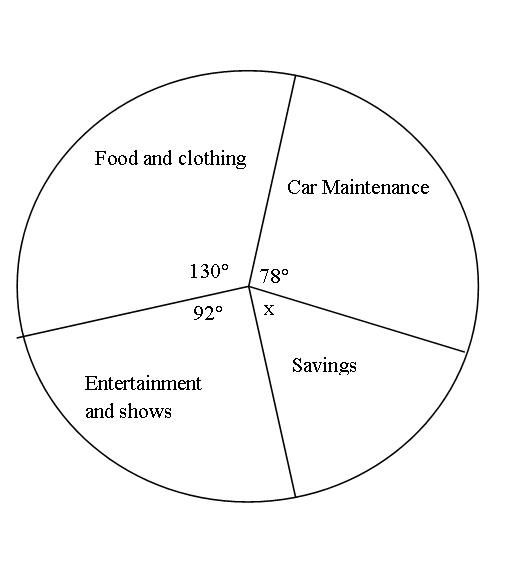Sign in# 2020 BECE: Examinable Mathematics Questions For BECE Candidates

MATHEMATICS Paper 2 Answer four questions only

1.(a) Find the solution set of 1 - 2x ≥ 2 - x. Illustrate your answer on a number line.

(b) Given the relation below(i) Express b in terms of x, y and w.

(ii) Find b when x = 1, w = 5 and y = -2

(c) Simplify2.(a)In the diagram above, triangle PQS and QSR are isosceles triangles. Also angle SRQ = 41°, calculate

(i) angle QSR

(ii) angle QPS

(b) Ben is twice as old as Esi who is then 5 years older than Kobina. If the sum of their ages is 51 years, find their ages.

(c) Evaluate 0.054 x 0.0021 x 0.00012 leaving your answer in standard form.

3.(a) Copy and complete the table below for the relation y - 2x - 1 = 0(b)(i) Using a scale of 2cm to 2 units on both axes, mark the x-axis from - 10 to 10 and y-axis from -8 to 18.

(ii) Plot the ordered pairs (x, y) on a graph sheet. use a ruler to join the points plotted

(i) x when y = 8

(ii) y when x = 5

(b) Factorize completely the expression: 8x2 - 8x - 4xy + 4y

4.(a) Using a pair of compasses and a ruler only, construct

(i) triangle XYZ such that /XY/ = 8cm, angle ZXY = 45° and XYZ = 60°.

(ii) a line parallel to /XY/ with an interval of 5cm. Label the point of intersection T and S respectively.

(b) Measure XT

(c) Amina sold an article and made a loss of 15%. If she bought the article at GH¢535.00, find the selling price?

5.(a) The pie chart below illustrate how a banker spends his monthly income. He saves GH¢ 2,500.00 every month.(i) Find the value of x

(ii) What is his monthly income?

(iii) How much does he spend on Food and clothing than Entertainments and shows?

(b) Calculate the percentage he spends on car maintenance.

(c) Add 232 base four to 312 four.

Content created and supplied by: Funni/Mathematics (via Opera News )

BECE Ben XYZ